085-1263404

### My Journal

All things Mathematical
21 Sep 2018
##### Question 1

A body travels in a straight line so that the distance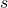feet travelled in timeseconds from a fixed point in the line of motion is shown by the following equation :-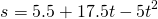.

Show that the body is travelling with uniform acceleration, find the value of the acceleration, and calculate the maximum distance from the fixed point reached by the body in the initial direction of motion.

##### Question 2

A simple pendulum hanging vertically inside a railway carriage, incline at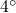to the vertical while the train is travelling round a circular curve on a horizontal track at a speed of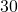miles per hour. Calculate the radius of the curve.

##### Question 3

Prove that in the case of a body moving in a straight line with simple harmonic motion the acceleration is proportional to the distance of the body from its mean position.

If the acceleration of such a body is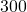feet per sec., per., when it is at a distance of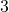feet from the mean position, find the period of vibration.

##### Question 4

The vertical load which is carried by one wheel of railway truck istons. The wheel itself weights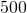lb. and is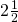feet in diameter.

If the centre of gravity of the wheel isinch from its geometric centre calculate the greatest and least pressures of the wheel on the track when the train is travelling at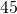miles per hour.

##### Question 5

A force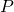inclined at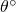to the horizontal is just able to drag a body of weight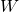slowly and uniformly along a rough horizontal surface.

Show thatis equal to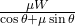.

(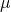represents the coefficient of friction.)

If the coefficient of friction is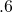, prove thatis a minimum when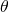is equal to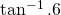.

##### Question 6

What time will be required to accelerate the speed of a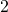ton motor car from miles per hour tomiles per hour, if the motion is resisted by a constant force oflb. per ton? It is assumed that the engine is working at the rate of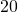horse-power at the beginning of this period and that it exerts a constant tractive effort on the wheels.

##### Question 7

A uniform pole leans against a vertical wall, the upper end touching the wall and the lower end resting on horizontal ground. The coefficient of friction at each end is. If the pole is on the point of slipping, find the value of the angle it makes with the horizontal.

##### Question 8

In the case of a group of particles whose individual masses, velocities, and accelerations are known, show how the velocity and acceleration of the centre of gravity of the system may be calcualted.

Two particles of mass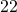grm. and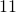grm. respectively are connected by a light, inextensible string passing over the edge of a horizontal table. The heavier mass rests on the table and the other hangs vertically. Neglecting frictional resistances find the tension in the string and the acceleration of the centre of gravity of the system.

##### Question 9

A body is projected from a height of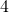feet so that it passes horizontally over the top of a building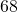feet high. The centre line of the building is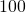feet from the point of projection of the body.

Calculate the magnitude and direction of the velocity of projection of the body. How far horizontally from the point of projection will the body hit the ground! [sic]

##### Question 10

Explain how the velocity of one body relative to another is obtained.

Two ships are sailing along straight courses with such constant, different velocities that they will collide unless their velocities are altered. Show that it would appear to a person on either of the ships, as if the other were always moving directly towards him.

##### Citation:

State Examinations Commission (2018). State Examination Commission. Accessed at: https://www.examinations.ie/?l=en&mc=au&sc=ru

Malone, D and Murray, H. (2016). Archive of Maths State Exams Papers. Accessed at: http://archive.maths.nuim.ie/staff/dmalone/StateExamPapers/

##### Licence:

“Contains Irish Public Sector Information licensed under a Creative Commons Attribution 4.0 International (CC BY 4.0) licence”.

The EU Directive 2003/98/EC on the re-use of public sector information, its amendment EU Directive 2013/37/EC, its transposed Irish Statutory Instruments S.I. No. 279/2005, S.I No. 103/2008, and S.I. No. 525/2015, and related Circulars issued by the Department of Finance (Circular 32/05), and Department of Public Expenditure and Reform (Circular 16/15 and Circular 12/16).

Note. Circular 12/2016: Licence for Re-Use of Public Sector Information adopts CC-BY as the standard PSI licence, and notes that the open standard licence identified in this Circular supersedes PSI General Licence No: 2005/08/01.